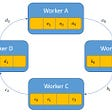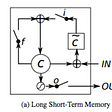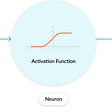# The AutoTest Framework Makes the Operator Alignment Task for Deep Learning Frameworks Easy

Written by Xiaoyu Zhang; Translated by Xiaozhen Liu, Chenyang Xu, Yakun Zhou

This article introduces OneFlow’s operator AutoTest framework to analyze how OneFlow perform the operator alignment task when we develop operators. This AutoTest Framework can also be easily ported to other deep learning training frameworks. The code is here.

Any deep learning training framework needs to verify the correctness of the operators. So, what is the general practice to verify the correctness of operators in deep learning frameworks? Taking Baidu’s deep learning framework PaddlePaddle as an example, the general practice is to call other standard libraries (e.g., call cudnn’s convolution to verify the correctness of the convolution operator, and call scipy’s erf to verify the correctness of the erf operator) or directly use the computational results of Numpy’s simulation (e.g., use Numpy for simulation to verify the correctness of the full operator). PyTorch also hard coding some test cases, which means comparing the standard answer of the fixed input cases with the result calculated by the operator to analyze the correctness of the operator.

There is nothing wrong with these approaches, but they require quite a lot of labor when writing tests and developers might forget some corner cases in the early stages of operator development. Taking OneFlow as an example, since the operator’s behavior is to align PyTorch, what kind of code can be used to fully verify the correctness of the transpose convolution operator in various cases? One approach would be to enumerate each parameter:

This approach is relatively comprehensive but has drawbacks. First, how to determine the upper bound of the enumeration? If a large upper bound is given, the verification time for the operator will be very long, which is not helpful in the CI process. If the upper bound is small, some corner cases may be overlooked, resulting in incomplete tests and increasing the risk of bugs in the operator.

Based on the above problems, we developed an operator AutoTest framework to align OneFlow operators with PyTorch operators. We also enriched the framework with some other features, which is easy enough to use. Later we will give a detailed introduction to this AutoTest framework.

The entire AutoTest framework consists of only 2 Python files: https://github.com/Oneflow-Inc/oneflow/blob/v0.6.0/python/oneflow/test_utils/automated_test_util/torch_flow_dual_object.py and https://github.com/Oneflow-Inc/oneflow/blob/v0.6.0/python/oneflow/test_utils/automated_test_util/generators.py. It can also be easily ported to any other deep learning training frameworks for use.

# 2. Usage of Operator AutoTest Framework

Before introducing the working principle, let’s look at the usage of AutoTest framework. Still taking the above transpose convolution operator as an example, after using the AutoTest framework, we can use the following code to perform the operator alignment test:

If you are familiar with PyTorch, you will notice that the above code is basically the same style as PyTorch’s code. Indeed, the AutoTest framework is like a higher level PyTorch, with the same interface as PyTorch. However, for a given input, it will run respectively through with OneFlow and PyTorch, record each tensor which is obtained during the run and record the values of the corresponding gradient tensors. Then, it will check whether the values and shapes of these tensors respectively generated by OneFlow and PyTorch are the same. Then, the automatic test work is done. For the details, we will talk about them later.

Let’s look at another test case on matmul operator.

Based on the `random_pytorch_tensor` method, we build two random tensors `x` and `y`, whose dimensions are `[m, k]` and `[k, n]` respectively. The values of these dimensions are generated randomly.

By running the above two test cases, the test automation framework will help us randomize the operators with various combinations of legal parameters. Then, it will run the PyTorch and OneFlow codes based on the input Tensor with identical values and types (one for PyTorch and one for OneFlow), and finish the automated testing of the operator. Since the usage of this test automation framework aligns with PyTorch’s, it is very easy to write test cases after we develop operators. We do not need to introduce other standard libraries or use Numpy to simulate the forward and reverse calculation process of the operator any more, which greatly liberates productivity.

With enough tests, there is a high probability that we can cover the samples where the OneFlow operator and PyTorch operator do not align. At this point, if there is corresponding reproducible example, we can determine whether there is a problem with the OneFlow operator.

# 3. Ideas behind Operator AutoTest Framework

After understanding how to use the AutoTest framework, let’s learn more about the idea behind the AutoTest framework. From the above usage, you might have guessed that the AutoTest framework is divided into two parts. One is how to generate random data, and the other is to run the program for the test part, record and compare the shapes and values of the tensors and the corresponding gradient tensors.

## 3.1 How to Generate Random Data

The random data here not only refers to the random input tensor, but also contains the operator’s property parameters. For example, `kernel_size=random(1, 4)` in the above deconvolution operator test case specifies that `kernel_size` will take values in the interval `[1, 4)`.

This part is realized in the file https://github.com/Oneflow-Inc/oneflow/blob/v0.6.0/python/oneflow/test_utils/automated_test_util/generators.py. First, let's see what interfaces are exported in this file:

These interfaces are classes that inherit from the `generator` base class to generate random data structures, where the data structures can be either built-in types such as `int` or custom data types such as `tensor`. All the random parameters of the AutoTest framework is generated based on these methods. Let's take a look at the `generator` base class implementation:

This class not only holds `_calc_value`, `value`, `eval` functions related to values, but also holds the `size` function that shows the number of generated data. In addition, the class also holds a series of magic functions, so that different `generator` subclasses can be combined with each other, which improves the flexibility of test automation framework. Finally,it has a `to` member function, which is overridden by classes that inherit from the `generator` base class, determining the numeric type of this random data structure.

All `generator` derived classes inherit from the `generator` base class and override `__init__`, `__calc_value`, `size`, `_to`, and other member functions. For example, the derived class of the `generator` of `nothing` directly overrides the `_calc_value` function and returns an entity of a class that does nothing in it.

For example, `random`, a derived class of `generator`, is defined as follows:

One thing to note here is that the `generator` derived class that holds the `annotation` attribute can use `to` to update the `annotation` attribute (such as the `random` class). Or it can ignore `annotation` and directly build a random result of the corresponding type in `_calc_value` (like the `random_device` class).

## 3.2 AutoTest Framework Core Implementation

AutoTest framework’s core implementation is in the file https://github.com/Oneflow-Inc/oneflow/blob/v0.6.0/python/oneflow/test_utils/automated_test_util/torch_flow_dual_object.py. The last 2 lines of code are:

`torch_original` in `torch = GetDualObject("", torch_original, flow)` represents the original PyTorch framework, while the `torch` obtained using `GetDualObject` represents a wrapping of the original PyTorch and OneFlow into a high level PyTorch. Therefore, the most critical implementation is `GetDualObject` function. Let's not focus on what this function is doing but on what it returns. Looking at the code, we can see that this function returns a `DualObject` class object. Let's examine this class first:

The class object name and the pytorch/oneflow objects are passed in `__init__`, and `torch_original` and `flow` are passed in when exporting the high level PyTorch, `pytorch_tensor` and `oneflow_tensor` is passed in when exporting `random_pytorch_tensor` interface. Let's take a look at the implementation of the `random_pytorch_tensor` function:

It can be seen that it obtains an object by calling `GetDualObject`, just like the implementation of exporting high level PyTorch. Going back to the implementation of `DualObject` class, we can see that the lists `dual_modules_to_test` and `dual_objects_to_test` are used to record the nn.Module and tensor objects of OneFlow and PyTorch, respectively. In addition, `DualObject` class overrides the magic method `__getattr__`. Let's take flatten as an example to see what attributes this magic method gets from the AutoTest program:

Then, let’s look at the printout of the key in `__getattr__`:

It can be seen that `nn.Module` or other functions of PyTorch or OneFlow in the test program modified by `autotest()` decorator override this method. It takes the parameters and properties of these nn.Module or other functions and returns a new `DualObject` object using `GetDualObject`. We can print what the `DualObject` object corresponding to `Flatten` is:

`GetDualObject` generates a `DualObject` object based on the incoming `Pytorch`,`OneFlow` objects and their names. `GetDualObject` function rewrites `__call__` magic function of the original PyTorch and OneFlow objects for the high level PyTorch, and returns a `DualObject` object.

This process also involves skipping some magic functions that don’t need attention and checking that the properties of the incoming objects are legal and binding specific types to the random data generated by the `generator` inheritance class based on the types of the default parameters of the nn.Module and other API (done in `get_args` function).

There is also a special sentence for the Tensor method because the Tensor method is called differently (via `getattr`) than other Modules and functions (via `__call__`).

That’s the implementation for `GetDualObject`. The related link is here: https://github.com/Oneflow-Inc/oneflow/blob/v0.6.0/python/oneflow/test_utils/automated_test_util/torch_flow_dual_object.py#L195-L401.

Finally, let’s look at the implementation of `autotest()` decorator:

This decorator’s `res = f(test_case)` will execute this decorator-modified autotest program, which will run PyTorch and OneFlow programs separately for a given input to obtain all intermediate output tensors, including the gradients of the tensor, and record them to the list `dual_modules_to_test`.

Then iterate through each tensor in this list and compare the values and the shape to see if they are the same. The comparison function is implemented on: https://github.com/Oneflow-Inc/oneflow/blob/v0.6.0/python/oneflow/test_utils/automated_test_util/torch_flow_dual_object.py#L565-L599.

The principle is to get the tensor’s numpy data for comparison. The `autotest()` decorator can also adjust several parameters, control whether the test is executed in reverse, the number of executions and the accuracy threshold for the final result comparison.

# 4. Automatically Generating Buggy Programs and Data

The above describes the principle and the usage AutoTest framework. Next, we will show how to get the program which can reproduce bugs and the corresponding input tensors and parameters based on the AutoTest framework. The principle is simple, which is to record the APIs used in the `GetDualObject` process and put them together to get a complete program. Here is a demonstration of how it works in CI: https://github.com/Oneflow-Inc/oneflow/runs/4760189461?check_suite_focus=true.

This example shows that `conv_transpose2d` operator of OneFlow and `conv_transpose2d` operator of PyTorch are not aligned under a certain case during a certain CI process. So CI also outputs the corresponding reproduction code and data when reporting this error, which can facilitate the framework developer to locate and determine.

In addition, this AutoTest framework is not only responsible for testing Eager operators, but also supports a variety of cases such as nn.Graph and Eager Consistent, which greatly facilitates framework developers.

# 5. Summary

This article introduces OneFlow’s operator AutoTest framework, which helps you more gracefully do operator alignment task in deep learning. It makes it easy for developers and users to write test programs like writing PyTorch. The AutoTest framework is flexible and easy to use, and you are welcome to learn about it and use it.

Related articles：

Also, welcome to join our Discord group to discuss and ask OneFlow related questions, and connect with OneFlow contributors and users all around the world.

--

--

--

## More from OneFlow

OneFlow is a performance-centered and open-source deep learning framework.

Love podcasts or audiobooks? Learn on the go with our new app.

## Finetuning BERT using ktrain for Disaster Tweets Classification## Manage your machine learning lifecycle with MLflow in Python## Visual intuition on ring-Allreduce for distributed Deep Learning## Taming the Hyper-Parameters of Mask RCNN## Distributed Training on Edge Devices: Communication compression## Hierarchical Multiscale Recurrent Neural Networks## CNN Feature Extraction: Between ReLU, Tanh and Sigmoid.## OneFlow

OneFlow is a performance-centered and open-source deep learning framework.

## How the DataLoader of OneFlow Works## Sky Computing: Accelerating Geo-distributed Computing in Federated Learning## Overview — Video Swin Transformer## Speed UP Inference on OVHcloud AI Training( PART 1)